400 600 7833    0453-6222286   137 6666 1299

# 干货 | 水处理常用计算公式汇总

1.栅隙

(1)水泵前格栅栅条间隙应根据水泵要求确定。

(2) 废水处理系统前格栅栅条间隙，应符合下列要求：最大间隙40mm，其中人工清除25~40mm，机械清除16~25mm。废水处理厂亦可设置粗、细两道格栅，粗格栅栅条间隙50~100mm。

(3) 大型废水处理厂可设置粗、中、细三道格栅。

(4) 如泵前格栅间隙不大于25mm，废水处理系统前可不再设置格栅。

2.栅渣

(1) 栅渣量与多种因素有关，在无当地运行资料时，可以采用以下资料。

(2) 栅渣的含水率一般为80%，容重约为960kg/m3。

(3) 在大型废水处理厂或泵站前的大型格栅（每日栅渣量大于0.2m3)，一般应采用机械清渣。

3.其他参数

(1) 过栅流速一般采用0.6~1.0m/s。

(2) 格栅前渠道内水流速度一般采用0.4~0.9m/s。

(3) 格栅倾角一般采用45°~75°，小角度较省力，但占地面积大。

(4) 机械格栅的动力装置一般宜设在室内，或采取其他保护设备的措施。

(5) 设置格栅装置的构筑物，必须考虑设有良好的通风设施。

(6) 大中型格栅间内应安装吊运设备，以进行设备的检修和栅渣的日常清除。

1.平面格栅设计计算

(1) 栅槽宽度B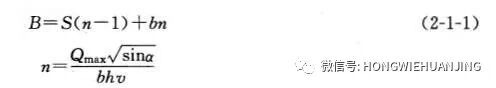(2) 过栅水头损失如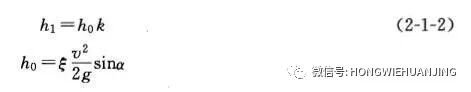(3) 榭后槽总高H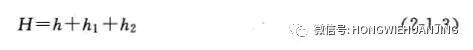(4) 栅槽总长L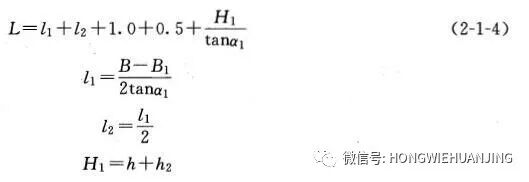(5)每日栅渣量W1.基底压力计算

(1)水池自重Gc计算

(2)池内水重Gw计算

(3)覆土重量计算

(4)活荷载作用Gh

(5)基底压力Pk

=(945.00+721.50+279.50+45.50+119.00)/42.500= 49.66 kN/m2

2.修正地基承载力

(1)计算基础底面以上土的加权平均重度rm

rm=[1.000×(20.00-10)+2.000×18.00]/3.000

= 15.33 kN/m3

(2)计算基础底面以下土的重度r

(3)根据基础规范的要求，修正地基承载力:

fa = fak + ηb γ(b - 3) + ηdγm(d - 0.5)

= 100.00+0.00×10.00×(5.000-3)+1.00×15.33×(3.000-0.5)

= 138.33 kPa

3.结论

Pk=49.66 <fa=138.33 kPa, 地基承载力满足要求。

Gk/F=1270.00/425.00=2.99 > Kf=1.05, 抗浮满足要求。

1.顶板荷载计算:

Qt = 1.20×P1 + 1.27×Ph= 7.91 kN/m2

Qte = P1 + 0.40×Ph= 5.60 kN/m2

2.池壁荷载计算: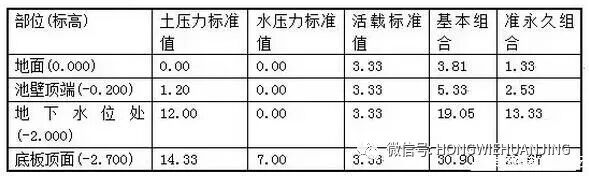3.底板荷载计算(池内无水，池外填土)：

Qb = (945.00×1.20+279.50×1.27+45.50×1.27+119.00×1.27×0.90)/42.500

= 39.59kN/m2

Qbe = (945.00+279.50+45.50×1.00+1.50×36.000×0.40+10.00×6.500×0.40)/42.500

= 31.00kN/m2

Q = 39.59-0.300×25.00×1.20= 30.59 kN/m2

Qe = 31.00-0.300×25.00

= 23.50 kN/m2

4.底板荷载计算(池内有水，池外无土):

Qb=[4.500×8.000×1.50×1.27+945.00×1.20+(3.900×7.400×2.500)×10.00×1.27]/42.500

= 49.86kN/m2

Q = 49.86-(0.300×25.00×1.20+2.500×10.00×1.27) = 9.11kN/m2

Qbe=[4.500×8.000×1.50×0.40+945.00+(3.900×7.400×2.500)×10.00]/42.500

= 39.72kN/m2

Qe=39.72-(0.300×25.00+2.500×10.00)

= 7.22kN/m2

1.弯矩正负号规则顶板：

2.荷载组合方式

1）.池外土压力作用(池内无水，池外填土)

2）.池内水压力作用(池内有水，池外无土)

3）.池壁温湿度作用(池内外温差=池内温度-池外温度)

B侧池壁内力：

1.池外填土，池内无水时，荷载组合作用弯矩表(kN·m/m)

2019年7月19日 16:12
?浏览量：0